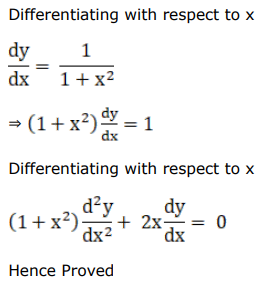# Solve this followingQuestion:

If $y=\tan ^{-1}$, show that $\left(1+x^{2}\right) \frac{d^{2} y}{d x^{2}}+2 x \frac{d y}{d x}=0$

Solution: